# SQuAD v2.0 Tokens Generated with WL

A list of isolated words and symbols from the SQuAD dataset, which consists of a set of Wikipedia articles labeled for question answering and reading comprehension

The Stanford Question Answering Dataset (SQuAD) consists of questions posed by crowd workers on a set of Wikipedia articles where the answer to every question is a segment of text, or span, from the corresponding reading passage. Unanswerable questions were added to the dataset for v2.0.

Modifications from the original dataset: Data marked "Training" in the ValidationRole field corresponds to the Training Set v2.0 subset of the original dataset. Data marked "Validation" in the ValidationRole field corresponds to the Dev Set v2.0 subset of the original dataset. The original dataset is 0-indexed; in order be accurate in the Wolfram Language, 1 was added to the value of "AnswerPosition", as the Wolfram Language is 1-indexed. This is a pre-processed form of the original dataset, where the pieces of text (Context, Questions and Answers) have been converted to a list of tokens with the following simple tokenization done in the Wolfram Language: SimpleTokenize[text_String] := DeleteCases[StringTrim @ StringSplit[text, {WordBoundary, x:PunctuationCharacter :> x}], ""];. The answers are then labeled with the field AnswerSpans, which corresponds to the first and the last indices of the tokens in Context. Most of the labeling errors of the original dataset could be detected and corrected during the process of computing these spans, given the character positions and the expected answer content.

## Examples

### Basic Examples

Retrieve the resource:

 In:=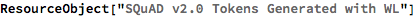Out=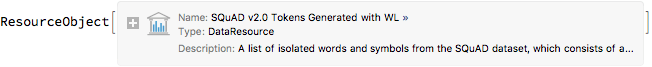Retrieve a sample of the dataset:

 In:=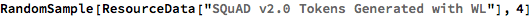Out=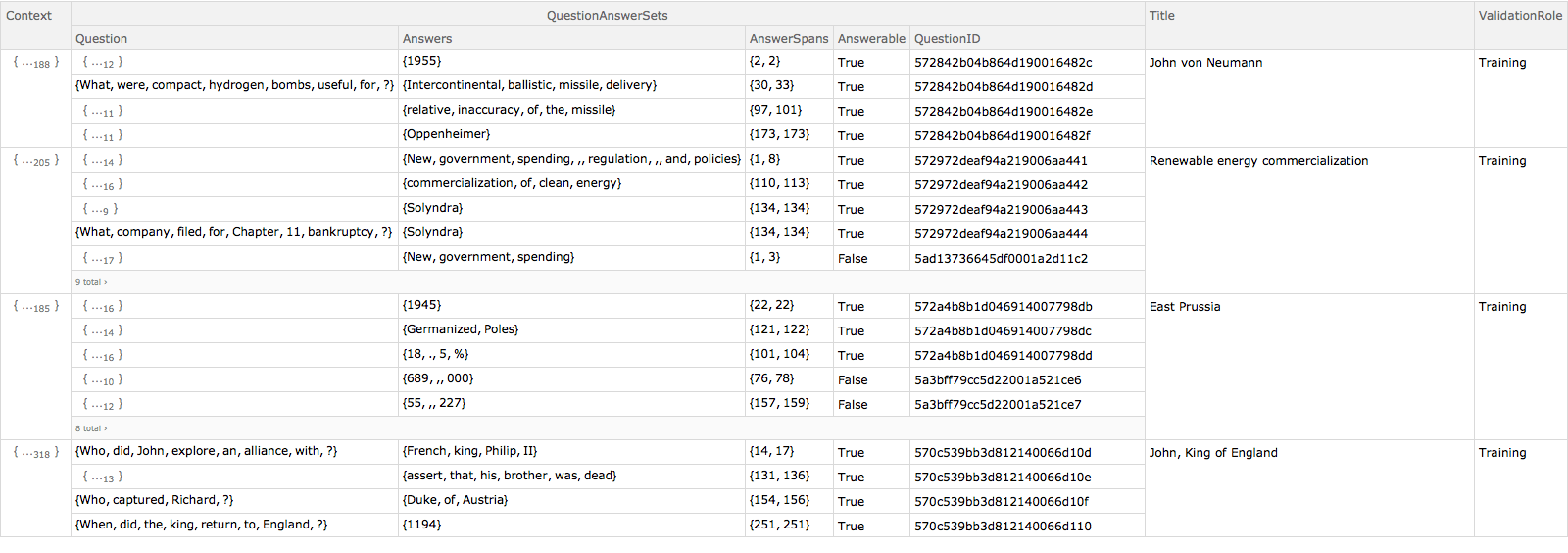### Working with Training and Validation Data

Obtain the first example from the training dataset:

 In:=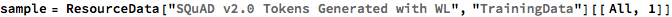Out=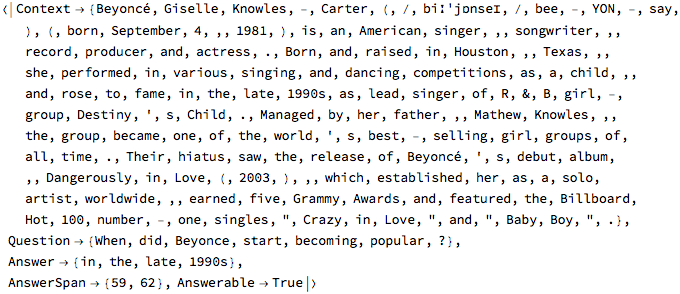View the question and answer associated with the passage:

 In:=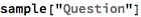Out=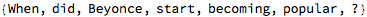In:=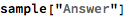Out=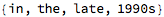"AnswerSpan" shows where the first word and the last word of the answer are located in the passage:

 In:=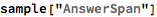Out=Test that the span between the starting position and the ending position is equivalent to the answer:

 In:=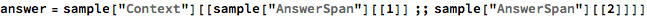Out=In:=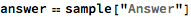Out=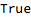In:=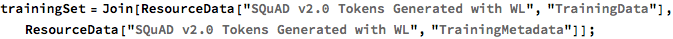There is a one-to-one correspondence between the data and the metadata; therefore, all lists will have the same length:

 In:=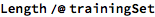Out=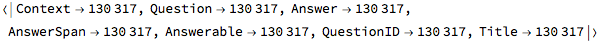View the joined data:

 In:=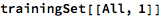Out=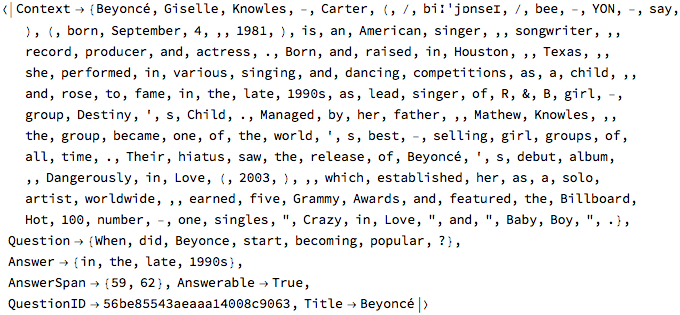### Dataset Size

Display the number of Wikipedia pages in the training and validation datasets:

 In:=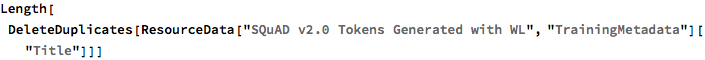Out=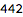In:=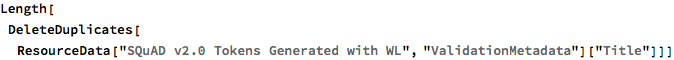Out=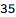Display the number of Wikipedia paragraphs in the training and validation datasets:

 In:=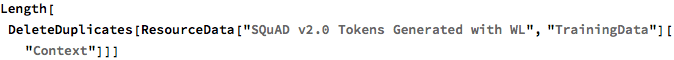Out=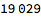In:=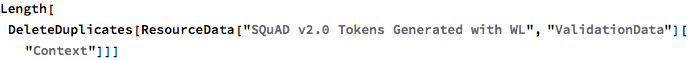Out=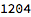Display the number of question/answer pairs in the training and validation datasets:

 In:=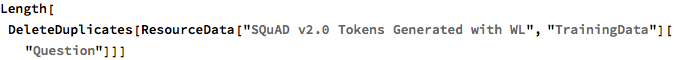Out=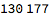In:=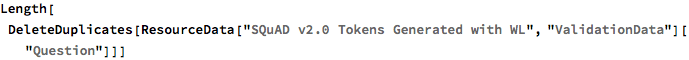Out=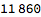Wolfram Research, "SQuAD v2.0 Tokens Generated with WL" from the Wolfram Data Repository (2019) https://doi.org/10.24097/wolfram.27825.data